## Another Twist

The algorithm we used to generate Pascal's Triangle can generalize still further. There is nothing special about addition; all we need for our algorithm to work is an operation with two inputs and one output, both from the same collection of possible inputs and outputs. When we add 1+2 to get 3, 1 and 2 are the "inputs" and 3 is the "output". 1, 2 and 3 are all from the same set of allowable numbers. For the original version of Pascal's Triangle, that set is the set of counting numbers. For our coloring scheme to work, we need to have a finite set of allowable inputs and outputs, and all outputs need to be possible inputs. We need the set to be closed under our operation. A set is closed under a given operation if whenever you perform the operation on members of the set, it returns another member of the set (as opposed to returning something not in the set). For example, if you add two counting numbers, you get another counting number so the counting numbers are closed under addition. If you add two numbers from the set {0, 1, 2, 3, 4} modulo 5, you get another number from the set {0, 1, 2, 3, 4}, so the set {0, 1, 2, 3, 4} is closed under mod 5 addition. However, if you divide two counting numbers, you may get a result that is not another counting number. For example 2 divided by 3 is 2/3 which is a fraction. So the counting numbers are not closed under division. Closure is absolutely required for our coloring scheme to work. When we combine two elements from our set, each of which is identified with a color, we must have another element of our set also identified with a color. An operation which takes two inputs from a given set and returns another element of the set is called a binary operation on the set. So we need a finite set and a binary operation on the set. There are some additional properties which are desirable:

1. Associativity: Many operations with which you are familiar are associative. If you add three to four to get seven, then add five, you get twelve. On the other hand if you add four to five to get nine, then add three to nine, you also get twelve. In other words, (3+4)+5=3+(4+5); it doesn't matter which two you associate first. This does not work for every operation. For example, if you divide twenty by ten to get two and then divide two by two, you get one. On the other hand if you divide ten by two to get five, then divide twenty by five, you get four, not one. (20/10)/2 does not equal 20/(10/2). It does matter which ones you associate first. To generalize this idea we say that an operation is associative if whenever you perform the operation on two elements, and then perform the operation again on the result of the first operation and a third element, you get the same result as you would if you performed the operation on the second element and the third element and then performed it again on the first element and the result of operating on the second and third. That's hard to follow, let us try it this way: an operation * on a set A is associative if for any elements a, b, and c of A:
(a*b)*c = a*(b*c)
Note . . .

2.

3. Identity: With addition on the integers, you have a zero element. Zero plus any other number just gives you the other number. The same is true of any number plus zero. 0+5=5+0=5. Thus zero is the additive identity. The number one works similarly with multiplication; one is the multiplicative identity. If you multiply 1 by any number, then you just get the number back. If a set has an element which, whenever you operate on that element and any other element of the set or on any other element of the set and that element, the operation returns the other element; then the element with this property is called the identity element for the operation. In other words, given an operation * on a set A, if there exists an element e of A such that for all other elements a of A:
e*a = a*e = a
then e is the identity element. An operation with an identity is said to have the identity property. Again note . . .

4.

5. Inverses: Once you have an identity, you can talk about inverses. Remember the additive inverse of a number is its negative, since when you add a number and its negative you get zero, the additive identity. The multiplicative inverse of a number is its reciprocal, since a number times its reciprocal is one, the multiplicative identity. If we restrict ourselves to the whole numbers, every number has an additive inverse but numbers like 2 and 3 do not have multiplicative inverses since 1/2 and 1/3 are not whole numbers. If an operation has an identity and the set also has the following property: each element has an element with which it can be associated such that the result of applying the operation to two associated elements always returns the identity element, then the operation has the inverse property. Two associated elements are called inverses of each other. In other words, given an operation * on a set A with an identity e, if for every element a in A :
there exists an element a-1 in A such that
a*a-1 = a-1*a = e,
then a-1 is called the inverse of a and the operation * has the inverse property. One more time note . . .

6.

It is possible to perform our coloring scheme using sets with operations without these three properties. However, sets together with binary operations having these properties have led to an entire branch of mathematics called Group Theory, which is a subset of Abstract Algebra. Therefore, when we are interested in adding a new twist to our patterns, it is natural for us to start with sets and operations with these three properties. A set together with a binary operation having properties 1 through 3 is called a group. A  finite set with such an operation is called a finite group. You can construct a  PascGalois Triangle (the next twist on Pascal's Triangle) by first associating with every element in a finite group a different color, then placing one element down one side of the triangle and a, possibly different, element down the the other side of the triangle, and finally using the group operation, generally referred to as group multiplication, to generate the interior. Pascal's triangle mod n for any integer n is one kind of example of PascGalois Triangles since the integers, {0, 1, 2, . . . n-1} under addition mod n are a finite group. We generally give names to groups so that we can easily refer to them. The set {0, 1, 2, ..., n-1} using addition mod n is called ZnZn is also called the cyclic group of order n.( why cyclic?) Remember that we have seen the multiplication tables for some of these groups.

Here is another example: Consider the set of ordered pairs {(0,0), (1,0), (0,1), (1,1)} and the operation where you combine two members of the set by adding the first components mod 2 to get the first component of the result and adding the second components mod 2 to get the second component of the result. Here is the multiplication table for this operation:

 * (0,0) (0,1) (1,0) (1,1) (0,0) (0,0) (0,1) (1,0) (1,1) (0,1) (0,1) (0,0) (1,1) (1,0) (1,0) (1,0) (1,1) (0,0) (0,1) (1,1) (1,1) (1,0) (0,1) (0,0)
Notice that this set is really like two copies of Z2 pasted next to each other. When two sets are put together to form a new set of ordered pairs in this manner, we call it a cross product. So this example is just the cross product of Z2 with itself, denoted Z2x Z2. Notice that the operation involved is just the same operation from Z2, performed on each of the elements in the pair separately. Would you like to see the PascGalois Triangle for this set?

All of the groups that we have looked at so far have an additional property that we did not list above. That property is

• 4. Commutativity: An operation is commutative if the order of the two elements being operated on doesn't matter. In other words, an operation * on a set A is commutative if for any a and b in the set A:
a*b = b*a
Groups whose operations have this additional property are called commutative groups or more frequently abelian groups. Groups whose operations do not have this property are called non-commutative or non-abelian. One well-known class of non-abelian groups are the dihedral groups, Dn. To illustrate these, let us begin by looking at D3. The elements of D3 are movements of an equilateral triangle which result in the triangle's still looking the same as it did before you moved it. Such a movement is called a symmetry. For example, if you flip an equilateral triangle around any one of its altitudes, the triangle still looks the same. See the figure below. The pink lines and the labels on the vertices are to help you to see what is going on but are not part of the triangle itself. Therefore, we do not say that the triangle looks different if the only change in its appearance is in the positioning of the labels or the pink lines. There are six different symmetric movements of the triangle; three of these involve flipping the triangle across an altitude as follows: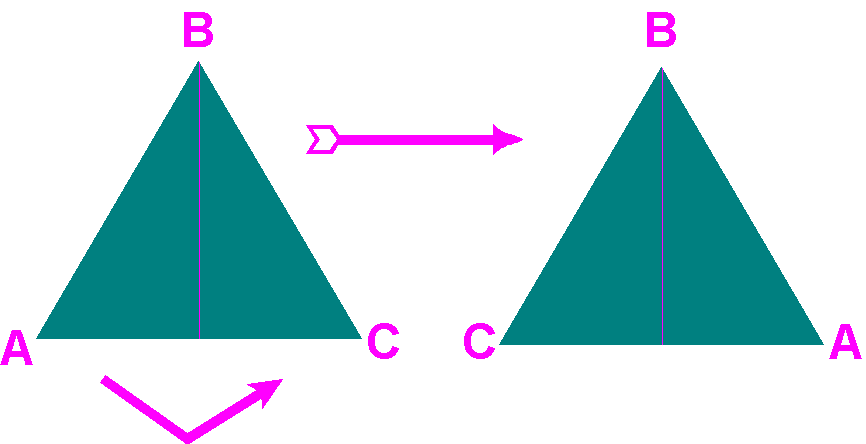Let's call this movement a.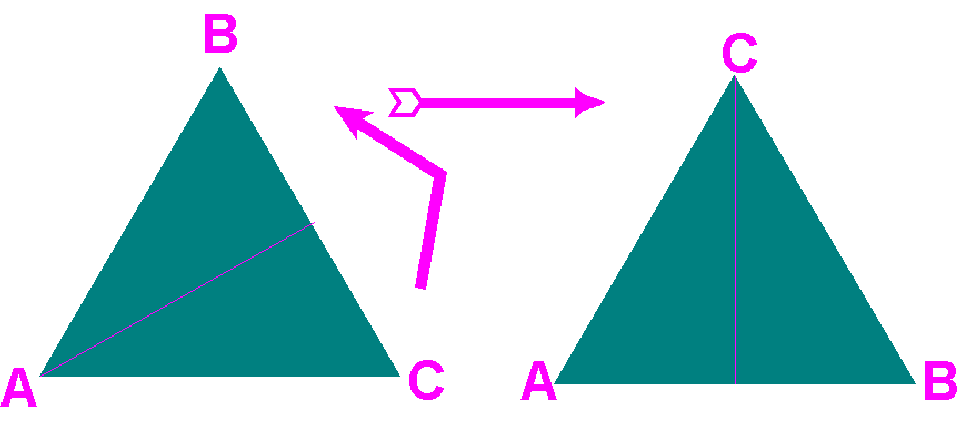Let's call this movement b.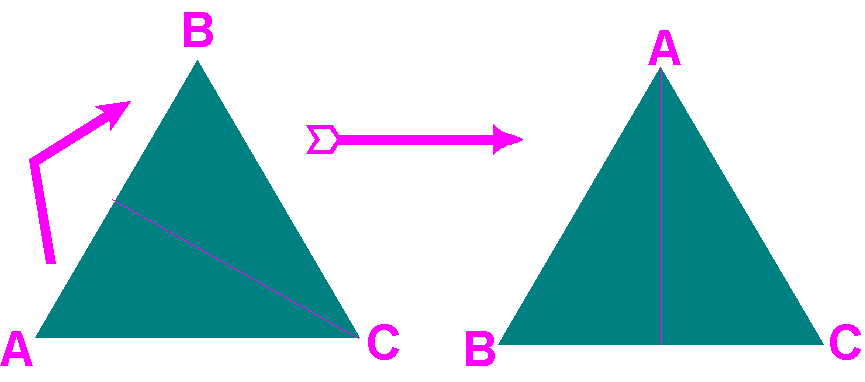Let's call this movement c.

The other three symmetric movements are rotations though 0 (Or 360) degrees, 120 degrees and 240 degrees as follows: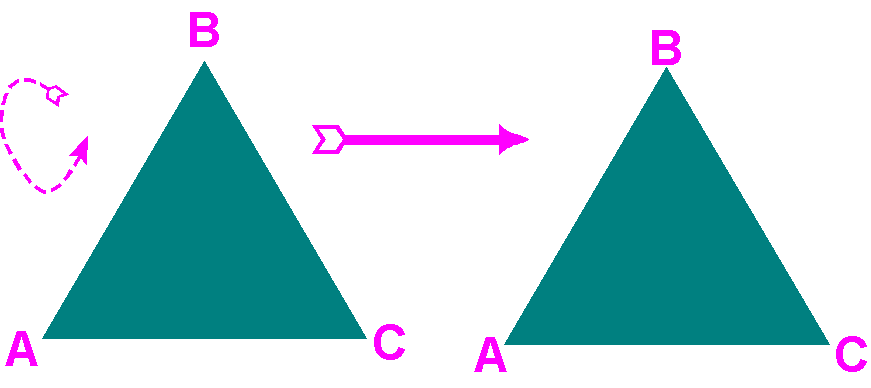Let's call this movement e;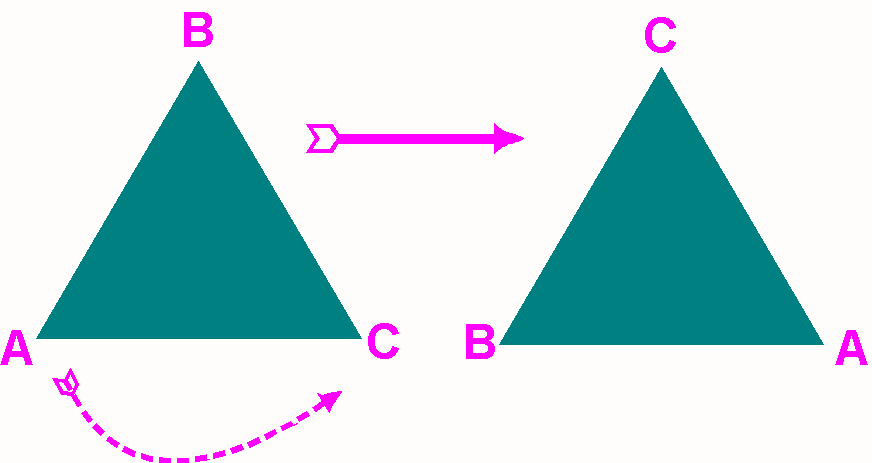Let's call this movement p.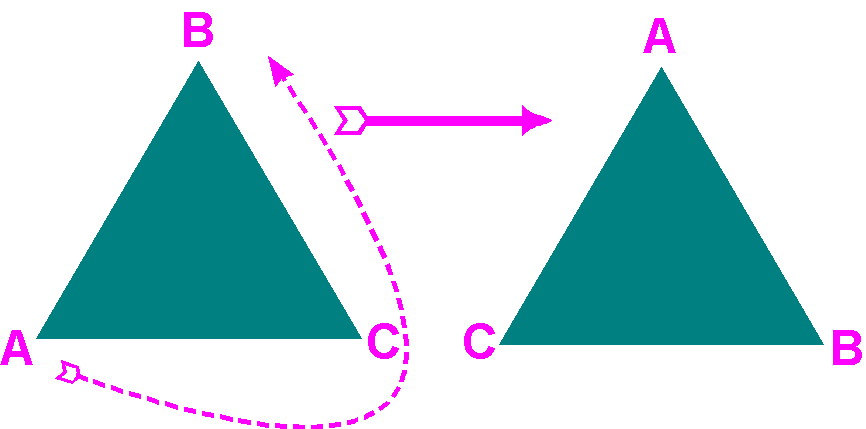Let's call this movement q.

The operation we will associate with this set of movements is just to perform the movements one after the other. For example if you perform movement a:followed by movement p: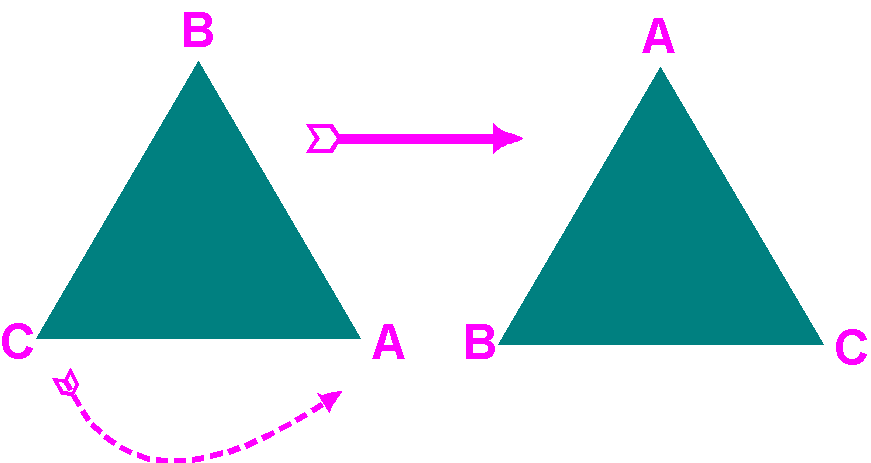you get the same result you would have gotten from performing movement c:So a*p=c.

We can try this with all combinations to get the multiplication table for this operation:

 * e p q a b c e e p q a b c p p q e b c a q q e q c a b a a c b e q p b b a c p e q c c b a q p e

Notice that properties 1 though 3 hold for this operation on this set of movements, but property 4 does not hold. For example, a*p = c, but p*a =b. They are not the same. Therefore, D3 is a non-abelian group. Let's see how the PascGalois triangles look for this group.

Of course, the equilateral triangle is not the only shape with symmetries. Although many shapes have symmetries, the easiest family of shapes to study in this manner are the regular polygons. Remember that a polygon is a many sided figure (where the sides are straight edges), and a regular polygon is one whose sides are all the same length. Thus an equilateral triangle is a regular polygon, a.k.a. a regular 3-gon, a square is a regular 4-gon, and the U.S. Pentagon is built in the shape of a regular 5-gon. A square has eight symmetries. You can flip it about either of its two diagonals or about either of its two altitudes or you can rotate it through 0, 90 180 or 270 degrees (remember that a rotation through 360 degrees is the same as a rotation through 0 degrees). So there are 4 flips and 4 rotations that are symmetries for the regular 4-gon. Thus D4 has 8 elements. Similarly, there are n flips and n rotations that are symmetries for a regular n-gon. The rotations are through multiples of 360/n degrees and the flips are across axes formed as follows: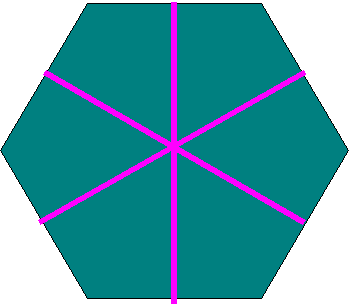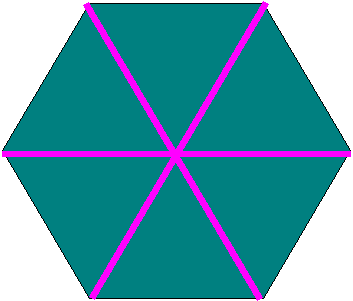•  if n is even: join the midpoints of sides that are across from each other or join corners that are across from each other.

•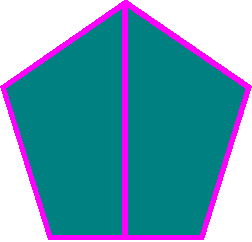• if n is odd join each corner to the midpoint of the opposite side.

Thus the symmetries of a regular n-gon will form a group with 2n elements and we call the group Dn. We define the multiplication in the same way we did in the D3 case. Again performing a flip followed by another flip results in a rotation, performing a rotation followed by another rotation results in a rotation, and performing either a flip then a rotation or a rotation then a flip, results in a flip. (You can verify this by cutting out a regular hexagon and a regular pentagon, labeling the corners, and flipping and rotating.) It is possible to generate multiplication tables and thus PascGalois triangles for these groups. Then it is interesting to see what the triangles look like.